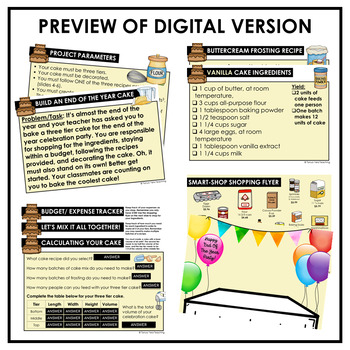# End of Year Activities Math Project Based Learning Fractions4th - 6th, Homeschool
Subjects
Standards
Resource Type
Formats Included
• PDF
•Google Apps™
Pages
20 pagesThe Teacher-Author indicated this resource includes assets from Google Workspace (e.g. docs, slides, etc.).

### Description

This resource is perfect for an end of the year math activity for your students to complete. Packed with engaging activities, your students will be asked to follow recipes to bake a three tier cake.

Aligned to curriculum, students will review key skills from the year and in the end create a three-dimensional cake to celebrate! This project based learning activity is just what you need to get thru the end of the year!

This activity covers the following math skills:

➥ Multiplying fractions by whole numbers

➥ Converting measurement

➥ Volume

Teacher directions and answer keys are included!

Interested in other PBL activities? Check out this one which focuses on creating angles using a protractor!

Project Based Learning Activity Installing Security Cameras PBL Activity Math

Total Pages
20 pages
Included
Teaching Duration
3 days
Report this Resource to TpT
Reported resources will be reviewed by our team. Report this resource to let us know if this resource violates TpT’s content guidelines.

### Standards

to see state-specific standards (only available in the US).
Understand addition and subtraction of fractions as joining and separating parts referring to the same whole.
Decompose a fraction into a sum of fractions with the same denominator in more than one way, recording each decomposition by an equation. Justify decompositions, e.g., by using a visual fraction model. Examples: 3/8 = 1/8 + 1/8 + 1/8; 3/8 = 1/8 + 2/8; 2 1/8 = 1 + 1 + 1/8 = 8/8 + 8/8 + 1/8.
Add and subtract mixed numbers with like denominators, e.g., by replacing each mixed number with an equivalent fraction, and/or by using properties of operations and the relationship between addition and subtraction.
Solve word problems involving addition and subtraction of fractions referring to the same whole and having like denominators, e.g., by using visual fraction models and equations to represent the problem.
Apply and extend previous understandings of multiplication to multiply a fraction by a whole number.